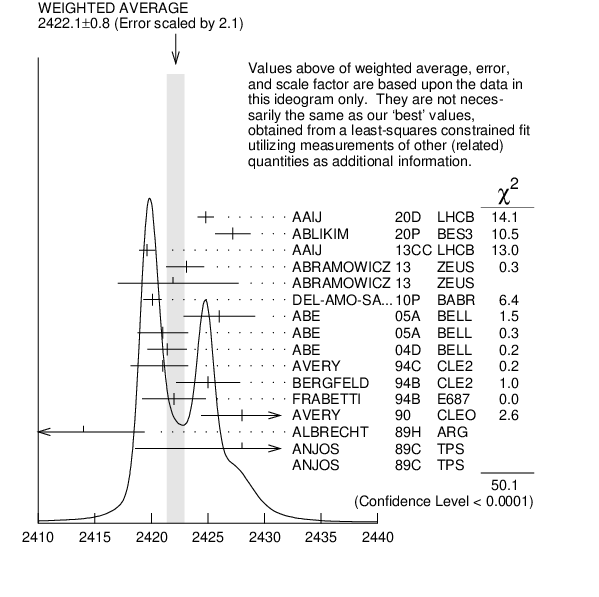${{\boldsymbol D}_{{1}}{(2420)}}$ MASS

The fit includes ${{\mathit D}^{\pm}}$, ${{\mathit D}^{0}}$, ${{\mathit D}_{{s}}^{\pm}}$, ${{\mathit D}^{*\pm}}$, ${{\mathit D}^{*0}}$, ${{\mathit D}_{{s}}^{*\pm}}$, ${{\mathit D}_{{1}}{(2420)}^{0}}$, ${{\mathit D}_{{2}}^{*}{(2460)}^{0}}$, and ${{\mathit D}_{{s1}}{(2536)}^{\pm}}$ mass and mass difference measurements.
VALUE (MeV) EVTS DOCUMENT ID TECN CHG  COMMENT
 $\bf{ 2422.1 \pm0.6}$ OUR FIT  Error includes scale factor of 1.7.
 $\bf{ 2422.1 \pm0.8}$ OUR AVERAGE  Error includes scale factor of 2.1. See the ideogram below.
$2424.8$ $\pm0.1$ $\pm0.7$ 79k 1
 2020 D
LHCB 0 ${{\mathit B}^{-}}$ $\rightarrow$ ${{\mathit D}^{*+}}{{\mathit \pi}^{-}}{{\mathit \pi}^{-}}$
$2427.2$ $\pm1.0$ $\pm1.2$ 4207
 2020 P
BES3 + ${{\mathit e}^{+}}$ ${{\mathit e}^{-}}$ $\rightarrow$ ${{\mathit D}^{+}}{{\mathit D}^{-}}{{\mathit \pi}^{+}}{{\mathit \pi}^{-}}$
$2419.6$ $\pm0.1$ $\pm0.7$ 210k
 2013 CC
LHCB 0 ${{\mathit p}}$ ${{\mathit p}}$ $\rightarrow$ ${{\mathit D}^{*+}}{{\mathit \pi}^{-}}{{\mathit X}}$
$2423.1$ $\pm1.5$ ${}^{+0.4}_{-1.0}$ 2.7k 2
 2013
ZEUS 0 ${{\mathit e}^{\pm}}$ ${{\mathit p}}$ $\rightarrow$ ${{\mathit D}^{(*)+}}{{\mathit \pi}^{-}}{{\mathit X}}$
$2421.9$ $\pm4.7$ ${}^{+3.4}_{-1.2}$ 759 3
 2013
ZEUS + ${{\mathit e}^{\pm}}$ ${{\mathit p}}$ $\rightarrow$ ${{\mathit D}^{(*)0}}{{\mathit \pi}^{+}}{{\mathit X}}$
$2420.1$ $\pm0.1$ $\pm0.8$ 103k
 2010 P
BABR 0 ${{\mathit e}^{+}}$ ${{\mathit e}^{-}}$ $\rightarrow$ ${{\mathit D}^{*+}}{{\mathit \pi}^{-}}{{\mathit X}}$
$2426$ $\pm3$ $\pm1$ 151
 2005 A
BELL 0 ${{\mathit B}^{-}}$ $\rightarrow$ ${{\mathit D}^{0}}{{\mathit \pi}^{+}}{{\mathit \pi}^{-}}{{\mathit \pi}^{-}}$
$2421$ $\pm2$ $\pm1$ 124
 2005 A
BELL + ${{\overline{\mathit B}}^{0}}$ $\rightarrow$ ${{\mathit D}^{+}}{{\mathit \pi}^{+}}{{\mathit \pi}^{-}}{{\mathit \pi}^{-}}$
$2421.4$ $\pm1.5$ $\pm0.9$ 4
 2004 D
BELL 0 ${{\mathit B}^{-}}$ $\rightarrow$ ${{\mathit D}^{*+}}{{\mathit \pi}^{-}}{{\mathit \pi}^{-}}$
$2421$ ${}^{+1}_{-2}$ $\pm2$ 286
 1994 C
CLE2 0 ${{\mathit e}^{+}}$ ${{\mathit e}^{-}}$ $\rightarrow$ ${{\mathit D}^{*+}}{{\mathit \pi}^{-}}$ X
$2425$ $\pm2$ $\pm2$ 146
 1994 B
CLE2 + ${{\mathit e}^{+}}$ ${{\mathit e}^{-}}$ $\rightarrow$ ${{\mathit D}^{*0}}{{\mathit \pi}^{+}}$ X
$2422$ $\pm2$ $\pm2$ 51
 1994 B
E687 0 ${{\mathit \gamma}}$ ${}^{}\mathrm {Be}$ $\rightarrow$ ${{\mathit D}^{*+}}{{\mathit \pi}^{-}}$ X
$2428$ $\pm3$ $\pm2$ 279
 1990
CLEO 0 ${{\mathit e}^{+}}$ ${{\mathit e}^{-}}$ $\rightarrow$ ${{\mathit D}^{*+}}{{\mathit \pi}^{-}}$ X
$2414$ $\pm2$ $\pm5$ 171
 1989 H
ARG 0 ${{\mathit e}^{+}}$ ${{\mathit e}^{-}}$ $\rightarrow$ ${{\mathit D}^{*+}}{{\mathit \pi}^{-}}$ X
$2428$ $\pm8$ $\pm5$ 171
 1989 C
TPS 0 ${{\mathit \gamma}}$ ${{\mathit N}}$ $\rightarrow$ ${{\mathit D}^{*+}}{{\mathit \pi}^{-}}$ X
$2443$ $\pm7$ $\pm5$ 190
 1989 C
TPS + ${{\mathit \gamma}}$ ${{\mathit N}}$ $\rightarrow$ ${{\mathit D}^{0}}{{\mathit \pi}^{+}}{{\mathit X}^{0}}$
• • We do not use the following data for averages, fits, limits, etc. • •
$2420.5$ $\pm2.1$ $\pm0.9$ 3.1k 5
 2009
ZEUS 0 ${{\mathit e}^{\pm}}$ ${{\mathit p}}$ $\rightarrow$ ${{\mathit D}^{*+}}{{\mathit \pi}^{-}}{{\mathit X}}$
$2421.7$ $\pm0.7$ $\pm0.6$ 7.5k
 2006 A
CDF 0 1900 ${{\mathit p}}$ ${{\overline{\mathit p}}}$ $\rightarrow$ ${{\mathit D}^{*+}}{{\mathit \pi}^{-}}{{\mathit X}}$
$2425$ $\pm3$ 235 6
 1998 M
DLPH 0 ${{\mathit e}^{+}}{{\mathit e}^{-}}$
 1 From a full four-body amplitude analysis of the ${{\mathit B}^{-}}$ $\rightarrow$ ${{\mathit D}^{*+}}{{\mathit \pi}^{-}}{{\mathit \pi}^{-}}$ decay.
 2 From the combined fit of the ${{\mathit M}}$( ${{\mathit D}^{+}}{{\mathit \pi}^{-}}$ ) and ${{\mathit M}}$( ${{\mathit D}^{*+}}{{\mathit \pi}^{-}}$ ) distributions. and A$_{{{\mathit D}_{{2}}}}$ fixed to the theoretical prediction of $-1$.
 3 From the fit of the ${{\mathit M}}$( ${{\mathit D}^{0}}{{\mathit \pi}^{+}}$ ) distribution. The widths of the ${{\mathit D}_{{1}}^{+}}$ and ${{\mathit D}_{{2}}^{*+}}$ are fixed to 25 MeV and 37 MeV, and A$_{{{\mathit D}_{{1}}}}$ and A$_{{{\mathit D}_{{2}}}}$ are fixed to the theoretical predictions of 3 and $-1$, respectively.
 4 Fit includes the contribution from ${{\mathit D}_{{1}}^{*}{(2430)}^{0}}$.
 5 Calculated using the mass difference $\mathit m({{\mathit D}_{{1}}^{0}}$) $−$ $\mathit m({{\mathit D}^{*+}})_{PDG}$ reported below and $\mathit m({{\mathit D}^{*+}})_{PDG}$ = $2010.27$ $\pm0.17$ MeV. The 0.17 MeV uncertainty of the PDG mass value should be added to the experimental uncertainty of 0.9 MeV.
 6 No systematic error given.${{\mathit D}_{{1}}{(2420)}}$ mass (MeV)
References:
 AAIJ 2020D
PR D101 032005 Determination of quantum numbers for several excited charmed mesons observed in $B^- \to D^{*+} \pi^- \pi^-$ decays
 ABLIKIM 2020P
PL B804 135395 Study of $e^{+}e^{-} \to D^{+} D^{-} \pi^{+} \pi^{-}$ at center-of-mass energies from 4.36 to 4.60 GeV
 AAIJ 2013CC
JHEP 1309 145 Study of ${{\mathit D}_{{J}}}$ Meson Decays to ${{\mathit D}^{+}}{{\mathit \pi}^{-}}$, ${{\mathit D}^{0}}{{\mathit \pi}^{+}}$ and ${{\mathit D}^{*+}}{{\mathit \pi}^{-}}$ Final States in ${{\mathit p}}{{\mathit p}}$ Collisions
 ABRAMOWICZ 2013
NP B866 229 Production of the Excited Charm Mesons ${{\mathit D}_{{1}}}$ and ${{\mathit D}_{{2}}^{*}}$ at HERA
 DEL-AMO-SANCHEZ 2010P
PR D82 111101 Observation of New Resonances Decaying to ${{\mathit D}}{{\mathit \pi}}$ and ${{\mathit D}^{*}}{{\mathit \pi}}$ in Inclusive ${{\mathit e}^{+}}{{\mathit e}^{-}}$ Collisions near $\sqrt {s }$ = 10.58 GeV
 CHEKANOV 2009
EPJ C60 25 Production of Excited Charm and Charm-Strange Mesons at HERA
 ABULENCIA 2006A
PR D73 051104 Measurement of Mass and Width of the Excited Charmed Meson States ${{\mathit D}_{{1}}^{0}}$ and ${{\mathit D}_{{2}}^{*0}}$ at CDF
 ABE 2005A
PRL 94 221805 Observation of the ${{\mathit D}_{{1}}{(2420)}}$ $\rightarrow$ ${{\mathit D}}{{\mathit \pi}^{+}}{{\mathit \pi}^{-}}$ Decays
 ABE 2004D
PR D69 112002 Study of ${{\mathit B}^{-}}$ $\rightarrow$ ${{\mathit D}^{**0}}{{\mathit \pi}^{-}}$ ( ${{\mathit D}^{**0}}$ $\rightarrow$ ${{\mathit D}}{}^{(*)+}$ ${{\mathit \pi}^{-}}$ ) Decays
 ABREU 1998M
PL B426 231 First Evidence for a Charm Radial Excitation, ${{\mathit D}^{*'}}$
 AVERY 1994C
PL B331 236 Production and Decay of ${{\mathit D}_{{1}}{(2420)}^{0}}$ and ${{\mathit D}_{{2}}^{*}{(2460)}^{0}}$
 BERGFELD 1994B
PL B340 194 Observation of ${{\mathit D}_{{1}}{(2420)}^{+}}$ and ${{\mathit D}_{{2}}^{*}{(2460)}^{+}}$
 FRABETTI 1994B
PRL 72 324 Measurement of the Masses and Widths of $\mathit L$ = 1 Charm Mesons
 AVERY 1990
PR D41 774 P-wave Charmed Mesons in ${{\mathit e}^{+}}{{\mathit e}^{-}}$ Annihilation
 ALBRECHT 1989H
PL B232 398 Resonance Decomposition of the ${{\mathit D}^{*}{(2420)}^{0}}$ Through a Decay Angular Analysis
 ANJOS 1989C
PRL 62 1717 Observation of Excited Charmed Mesons